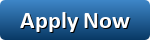## How banks calculate your home loan EMI

• Your EMI depends on three factors — the loan amount, the rate of interest and the loan tenure
• Banks use the daily reducing balance method for home loans, while some housing finance companies use the monthly method

Most people have multiple loans. As a result, the equated monthly instalment (EMI) outgo accounts for a major portion of our monthly expenditure, especially for those who have a home loan . And still, few of us take out the time to understand how banks arrive at the EMI number.

Your EMI constitutes the principal as well as the interest components. During initial years, the principal component is far less than the interest part, which is calculated on the outstanding balance of the principal.

## Interest payment

There are three ways in which a bank can charge interest— monthly reducing, annual reducing and daily reducing balance. Banks use the daily reducing balance for home loans, while some housing finance companies use the monthly method.

Under the daily method, the principal reduces on the day you pay the EMI. However, as EMI is paid monthly, there’s no difference in the effective interest rate, unless you prepay. In case of a prepayment, the outstanding principal will reduce on the day you make partial prepayment. So if you pay your EMI on the fifth of every month and prepay on the 10th, principal outstanding will reduce immediately in the daily method. Under the monthly method, the prepayment would be taken as on the fifth of the next month.

Your repayment over the tenor is shown in a loan amortisation schedule, a tabular presentation of how much interest and principal you pay and the outstanding principal at the end of every month.

## Calculating EMIs

The below example shows how banks calculate  home loan EMI:

The EMI depends on three factors—the loan amount, the rate of interest and the loan tenure. You can easily calculate the EMI using the PMT formula in Excel. For that, you’ll need three variables—the interest rate (rate), the loan period (nper) and the loan amount or the present value (PV). The rate of interest will be taken as monthly rate as EMIs are paid monthly. Therefore, if the interest rate is 10%, you need to divide it by 12. Also, the tenure (nper) will be the number of months. So, if your loan tenure is 20 years, the tenure will be 20 x 12 = 240 months. So, your EMI on a loan of ₹50 lakh at 10% interest rate and tenure of 20 years will be ₹48,251.

You can also use the mathematical formula

P*R*((1+R)^n)/(1-(1+R)^n)

where P is the principal outstanding, R is the monthly rate of interest and n is the number of monthly installments.

How banks calculate home emi : calculate here   EMI CALCULATOR